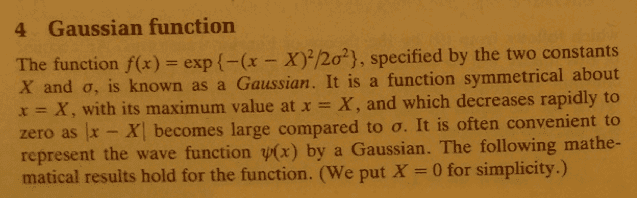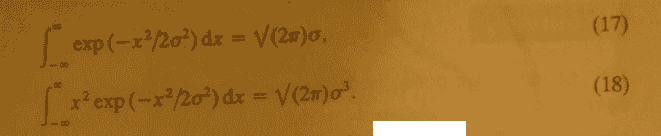# Gaussian function

g.lemaitre

## Homework Statement## The Attempt at a Solution

Looking at equations 17 and 18, I don't see how that follows. If you substitute infinity for x you're going to get infinity divided by some real number which is infinity

Hey g.lemaitre.

The first result is not intuitive and it is based on what is calle the error function or erf(x). The function does converge because e^(-x) when x gets really large goes quickly to 0.

http://en.wikipedia.org/wiki/Error_function

For the second one, you need to use a substitution of u = x^2. If you have done a year of calculus, this should be straight-forward.

Homework Helper
Gold Member
Looking at equations 17 and 18, I don't see how that follows. If you substitute infinity for x you're going to get infinity divided by some real number which is infinity

$e^{- \infty}=0$

Hey g.lemaitre.

The first result is not intuitive and it is based on what is called the error function or erf(x). The function does converge because e^(-x) when x gets really large goes quickly to 0.

There is no need for the error function when evaluating the first integral, just use the fact that $\int_{ - \infty }^{ \infty } f(x)dx = \int_{ - \infty }^{ \infty } f(y)dy$ to calculate the square of the integral, by switching to polar coordinates.

Do you mean ydx and xdy instead of f(x)dx f(y)dy? Sorry to nitpick but changing x to y is change a dummy variable change rather than a variable description change.

Homework Helper
Gold Member
Do you mean ydx and xdy instead of f(x)dx f(y)dy? Sorry to nitpick but changing x to y is change a dummy variable change rather than a variable description change.

The whole point is to exploit a change of the "dummy" integration variable, to transform the problem from one of finding a one-dimensional integral, to one of finding a two-dimensional integral, as the latter turns out to be easier:

$$\left( \int_{-\infty}^{\infty} f(x)dx \right)^2 = \left( \int_{-\infty}^{\infty} f(x)dx \right)\left( \int_{-\infty}^{\infty} f(y)dy \right) = \int_{-\infty}^{\infty} \int_{-\infty}^{\infty} f(x)f(y)dxdy$$

To see why it's easier, just switch to polar coordinates.

$$\left( \int_{-\infty}^{\infty} f(x)dx \right)^2 = \left( \int_{-\infty}^{\infty} f(x)dx \right)\left( \int_{-\infty}^{\infty} f(y)dy \right) = \int_{-\infty}^{\infty} \int_{-\infty}^{\infty} f(x)f(y)dxdy$$